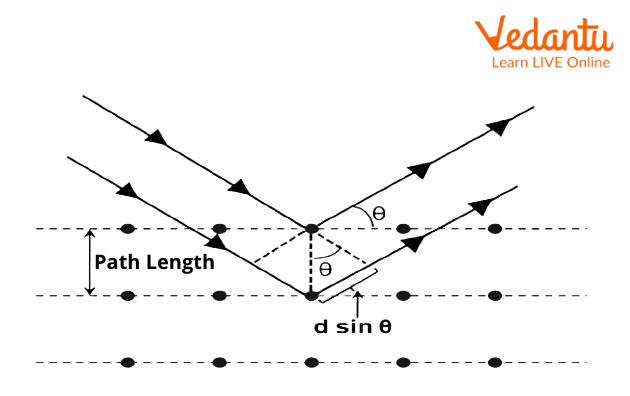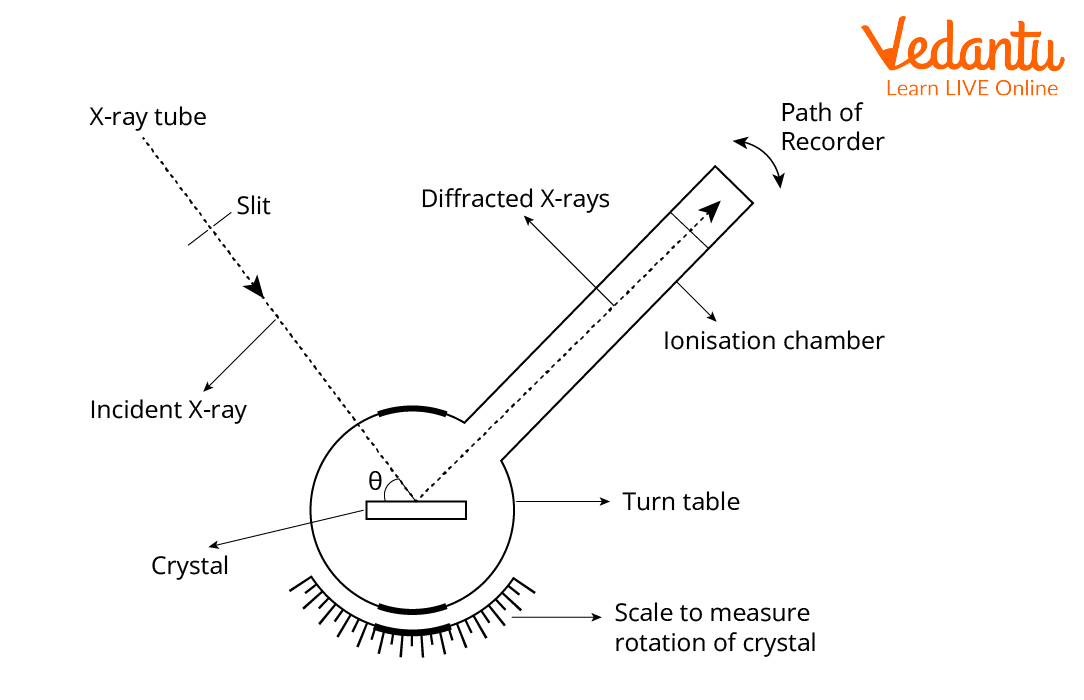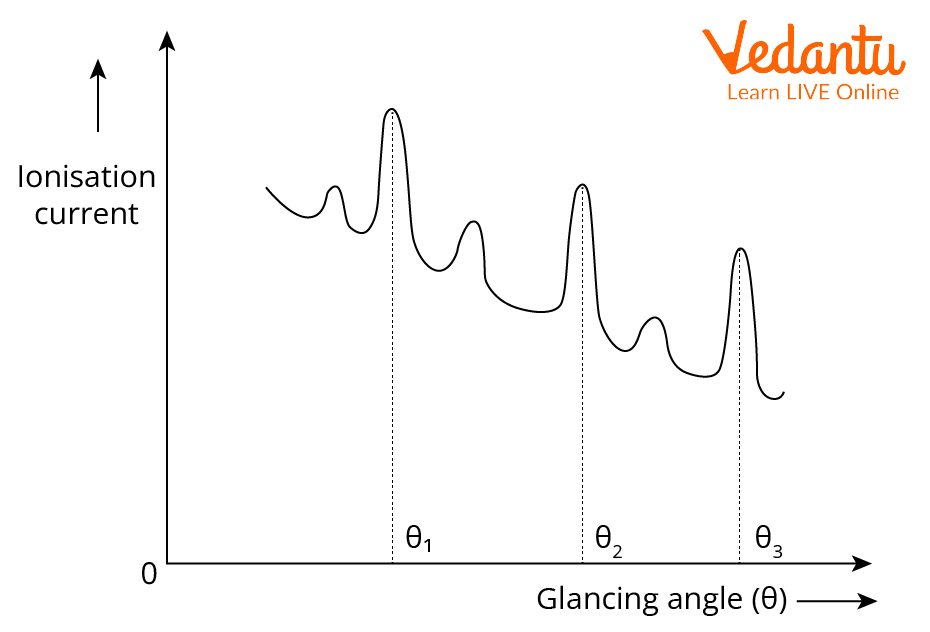Courses
Courses for Kids
Free study material
Offline Centres
More

# Bragg's LawLast updated date: 28th Nov 2023
Total views: 337.5k
Views today: 8.37k## An Overview of Bragg’s Law

The structures of crystals and molecules are usually being identified with the use of X-ray diffraction studies, which are explained by Bragg’s Law. The law explains the relationship between an X-ray light shooting into and its reflection off from the crystal surface.

It reveals the structure of the crystal we used. It is a simple but justifiably famous law, it brought a new scope to Crystallography. Bragg’s law made the study of the properties of various crystals easy. Using this, we can categorise the crystals into different classes.

## What is Bragg’s Law?

Lawrence Bragg found how to view the positions of atoms in solids. His discovery has had a giant effect on Chemistry, Biology, and mineralogy. Bragg confirmed how X-rays passing through a crystal acquire information permitting the crystal’s atomic shape to be deduced. X-ray records help scientists to construct 3-D fashions of the way atoms are organised in solids. Bragg’s discovery became arguably the greatest experimental leap forward in 20th-century science.

The law states that when the x-ray is incident on the surface of a crystal, with an angle of incidence $\theta$, it will reflect with the same angle of scattering. Also, constructive interference occurs under the condition when the path difference is equal to the whole number n of the wavelength. It is a special case of Laue diffraction, which determines the incoherent and coherent scattering. The waves from the crystal surface can interfere constructively or destructively. The analysis which is made as the result of the interference of waves is known as Bragg diffraction. We can write Bragg’s equation as follows:

$n\lambda=2dsin{\theta}$

Here

• n is an integer.

• $\lambda$ is the wavelength of the X-ray that incident on the crystal surface.

• d is the distance between the atomic layers.

• $\theta$ is the angle with which the X-ray incident on the crystal surface.

The law was first formulated by Lawrence Bragg, who is an English physicist. It came out from the surprising patterns produced on the crystalline solids when the x-ray is incident. Also, they found that at specific wavelengths and certain angles incidents produce sharp peaks of reflected radiation. Bragg interpreted the constructive interference geometrically such that the path difference is the multiple of the incident wavelength. The peaks that formed are known as Bragg’s peaks.

## Bragg’s Law Derivation

Consider a crystal that has parallel planes of ions that are spaced at a distance d apart. The conditions for a sharp peak in the intensity of the scattered radiations are as follows:

• The x-rays should be reflected by the ions in any one of the planes.

• The reflected rays that come out from the crystal’s successive planes should interfere constructively.Bragg’s Equation

The path difference is the phase shift. The constructive interference occurs when:

$\Delta=n\lambda$

From the diagram, we can write

$sin{\theta}=\frac{x}{d}$ and

$x= dsin{\theta}$

These criteria give the condition for constructive interference. That is, $2dsin{\theta}=n\lambda$ is the expression for Bragg’s law. Bragg’s law tells the angle at which the maximum diffraction intensity can occur.

## Bragg’s Spectrometer

It is used to study the crystals using x-rays. The apparatus consists of an X-ray tube from which x-ray beams fall on the crystal that is mounted on a rotating table. The table itself consists of a scale and vernier, from which we can measure the angle of incidence. There is an arm that rotates about the same axis as the crystal table. The rays reflected from the crystal enter the ionisation chamber, as a result of ionisation current flow occurs between the electrode, and the current is measured. It gives the intensity of the x-ray reflected. At certain angles ionisation current is high. From the graph, the glancing angles for different orders of reflection are measured. By knowing the angle and spacing of a crystal, we can easily find the wavelength of the X-rays.X-ray SpectrometerThe Ionisation Current Peaks at Specific Angles

## Applications of Bragg’s Law

As we know, Bragg’s law made a breakthrough in the field of crystallography. It has some useful applications.

1. In X-ray diffraction, the interplanar spacing of a crystal is used for the identification purposes.

2. It is useful for conducting the measurements of the wavelength of different families of crystals.

3. In X-ray fluorescence spectroscopy (XRS), the crystals with known interplanar spacings are used to analyse the crystals in the spectrometer.

## Interesting Fact

The principle of Bragg's law is applied within the construction of instruments like the Bragg spectrometer, which is commonly used to study the structure of crystals and molecules.

## Summary

Bragg’s law is widely used in crystallographic techniques. We have discussed about the brief history and analysis of Bragg’s law. The discovery of this law is a breakthrough in the Twentieth-century science field. It has enormous applications in the field of medical science, chemistry, Physics, etc. Bragg’s law basically shows the relation between the interplanar spacing and the angle of diffraction.

## FAQs on Bragg's Law

1. What Does Bragg's Law Explain?

In the subject Physics, Bragg's law, or even we can call it the Wulff–Bragg's condition is a special case of diffraction of Laue which gives the angles for incoherent and coherent scattering from a crystal lattice. When the X-rays fall on an atom they make the cloud of electronics that could  move, as does any electromagnetic wave, this whole process of analysis is called Bragg diffraction.

2.  In Bragg's Law What is the Order of Diffraction?

The law of Bragg's provides the condition for a plane of wave which is to be diffracted by a family of lattice planes: 2 d sin θ = n λ where the letter d which denotes the interplanar spacing, θ the angle between the vector wave of the incident plane wave denoted by k₀, and the lattice planes, λ is the wavelength and n letter is an integer, this is the order of the reflection.

3. How to Calculate d Spacing in Bragg's Law?

It can be described or calculated by the Bragg's law as following:

λ=2dsin where λ is for the wavelength of the X-ray beam that is 0.154nm. d is the letter which denotes distance between the adjacent that is GO layer or sheets, Ɵ is the diffraction of angle.

4. Mention the Difference Between Interference and Diffraction?

Interference is said to be a property which is originated by waves from two different coherent sources, whereas wavelets of the secondary originate from the same wave but occur from different parts so to produce a phenomenon which is termed as Diffraction.

5. What is an X-ray?

X-rays are electromagnetic radiations, similar to that of visible light. They have higher energy and can penetrate through almost all objects. They have a wavelength of order 0.01-10 nm. They have a great role in medical physics. When they pass through the human body, we generate images of tissues inside the body. The image thus formed is the shadows of the corresponding tissues. From the images, we can do further analysis. Those x-ray images obtained from the process are called radiographs.

6. What is the difference between diffraction and interference?

Interference could be defined as waves rising from 2 different sources, producing completely different wavefronts. Diffraction, on the other hand, are often termed as secondary waves that emerge from the various parts of the same wave. There are 2 different kinds of interference: proactive interference and retroactive interference. Fraunhofer and Fresnel are the 2 types of diffraction. The width of the fringes in interference is equal. The width of the fringes isn't equal in diffraction. The contrast between maxima and minima is extremely good whereas the contrast between maxima and minima is poor.

7. How is Bragg's law used in XRD?

XRD follows Bragg's law in that the reflected X-rays from different crystal layers with long range order undergo constructive interference. This causes high-intensity peaks within the spectrum. For materials without long range order like amorphous systems, no peaks are discovered. The Bragg’s law is helpful for measuring wavelengths and for determining the lattice spacings of crystals. To measure a specific wavelength, the radiation beam and the detector are both set at some arbitrary  angle θ. The angle is then changed till a powerful signal is received.